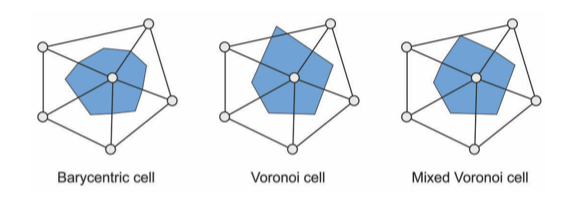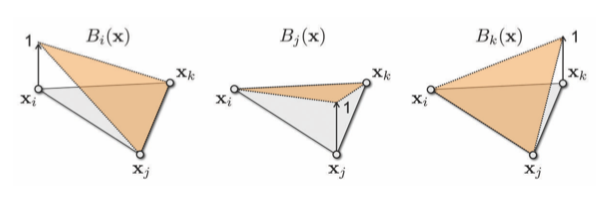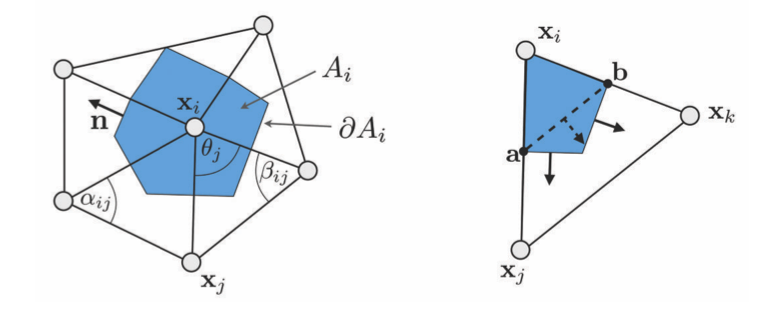Laplacian on Triangle Mesh | HyperPlane

## 预备内容

### 局部平均区域### 梯度

$f(\mathbf{u}) = f_iB_i(\mathbf{u}) + f_jB_j(\mathbf{u}) + f_kB_k(\mathbf{u})$$\nabla f(\mathbf{u}) = f_i \nabla B_i(\mathbf{u}) + f_j \nabla B_j(\mathbf{u}) + f_k \nabla B_k(\mathbf{u})$

$\nabla f(\mathbf{u}) = (f_j - f_i) \nabla B_j(\mathbf{u}) + (f_k - f_i) \nabla B_k(\mathbf{u})$

$\nabla B_i (\mathbf{u}) = \frac{(\mathbf{x}_k - \mathbf{x}_j)^{\perp}}{2A_T}$

$\nabla f(\mathbf{u}) = (f_j - f_i) \frac{(\mathbf{x}_i - \mathbf{x}_k)^{\perp}}{2A_T} + (f_k - f_i) \frac{(\mathbf{x}_j - \mathbf{x}_i)^{\perp}}{2A_T}$

## 离散拉普拉斯算子

### 均匀拉普拉斯

$\Delta f(v_i) = \frac{1}{|\mathcal{N}_1(v_i)|} \sum_{v_j \in \mathcal{N}_1(v_i)} (f_j - f_i)$

### 余切公式

$\intop_{A_i} \text{div} \mathbf{F}(\mathbf{u}) \text{d}A = \intop_{\partial A_i} \mathbf{F}(\mathbf{u}) \cdot \mathbf{n}(\mathbf{u}) \text{d}s$$\intop_{A_i} \Delta f(\mathbf{u}) \text{d}A = \intop_{A_i} \text{div} \nabla f(\mathbf{u}) \text{d}A = \intop_{\partial A_i} \nabla f(\mathbf{u})\cdot \mathbf{n}(\mathbf{u})\text{d}s$

\begin{aligned} \intop_{\partial A_i \cap T} \nabla f(\mathbf{u}) \cdot \mathbf{n}(\mathbf{u}) \text{d}s &= \nabla f(\mathbf{u}) \cdot (\mathbf{a} - \mathbf{b})^{\perp} \\ &= \frac{1}{2} \nabla f(\mathbf{u}) \cdot (\mathbf{x}_j - \mathbf{x}_k)^{\perp} \end{aligned}

\begin{aligned} \intop_{\partial A_i \cap T} \nabla f(\mathbf{u}) \cdot \mathbf{n}(\mathbf{u}) \text{d}s &= (f_j - f_i)\frac{(\mathbf{x}_i - \mathbf{x}_k)^{\perp} \cdot \mathbf{x}_j - \mathbf{x}_k)^{\perp}}{4A_T} \\ &+ (f_k - f_i)\frac{(\mathbf{x}_j - \mathbf{x}_i)^{\perp} \cdot \mathbf{x}_j - \mathbf{x}_k)^{\perp}}{4A_T} \end{aligned}

$\gamma_j$$\gamma_k$分别表示顶点$v_j$$v_k$的三角形内角。由于有

$A_T = \frac{1}{2} \sin \gamma_j \| \mathbf{x}_j - \mathbf{x}_i \| \| \mathbf{x}_j - \mathbf{x}_k \| = \frac{1}{2} \sin \gamma_k \| \mathbf{x}_i - \mathbf{x}_k \| \| \mathbf{x}_j - \mathbf{x}_k \|$

\begin{aligned} \cos \gamma_j &= \frac{( \mathbf{x}_j - \mathbf{x}_i ) \cdot ( \mathbf{x}_j - \mathbf{x}_k )}{\| \mathbf{x}_j - \mathbf{x}_i \|\| \mathbf{x}_j - \mathbf{x}_k \|} \\ \cos \gamma_k &= \frac{( \mathbf{x}_i - \mathbf{x}_k ) \cdot ( \mathbf{x}_j - \mathbf{x}_k )}{\| \mathbf{x}_i - \mathbf{x}_k \|\| \mathbf{x}_j - \mathbf{x}_k \|} \end{aligned}

$\intop_{\partial A_i \cap T} \nabla f(\mathbf{u}) \cdot \mathbf{n}(\mathbf{u}) \text{d}s = \frac{1}{2} (\cot \gamma_k (f_j - f_i) + \cot \gamma_j (f_k - f_i))$

$\intop_{A_i} \Delta f(\mathbf{u}) \text{d}A = \frac{1}{2} \sum_{v_j \in \mathcal{N}_i(v_i)} (\cot \alpha_{i,j} + \cot \beta_{i,j})(f_i - f_j)$

$\alpha_{i,j}$$\beta_{i,j}$$\mathbf{x}_i$$\mathbf{x}_j$的位置关系在上图有给出。

$\Delta f(\mathbf{u}) \coloneqq \frac{1}{2 A_i} \sum_{v_j \in \mathcal{N}_i(v_i)} (\cot \alpha_{i,j} + \cot \beta_{i,j})(f_i - f_j)$

## 矩阵化求解

### 矩阵化

$\Delta f(v_i) = w_i \sum_{v_j \in \mathcal{N}_1(v_i)} w_{ij} (f(v_j) - f(v_i))$

$\begin{pmatrix} \Delta f(v_1) \\ \vdots \\ \Delta f(v_n) \end{pmatrix}=\underbrace{DM}_L \begin{pmatrix} f(v_1) \\ \vdots \\ f(v_n) \end{pmatrix}$

$m_{i,j} = \begin{cases} -\sum_{v_k \in \mathcal{N}_1(v_i)} w_{ik}, &i = j \\ w_{ij}, &v_j \in \mathcal{N}_i(v_i) \\ 0, & \text{otherwise} \end{cases}$

$\Delta^k f(v_i) = w_i \sum_{v_j \in \mathcal{N}_1(v_i)} w_{ij} ( \Delta^{k-1} f(v_j) - \Delta^{k-1} f(v_i))$

$\mathbf{L}^k \mathbf{x} = \mathbf{b}$

### 矩阵性质

$\mathbf{M} (\mathbf{DM})^{k-1} \mathbf{x} = \mathbf{D}^{-1} \mathbf{b}$

$(-1)^k \mathbf{M} (\mathbf{DM})^{k-1} \mathbf{x} = (-1)^k \mathbf{D}^{-1} \mathbf{b}$

## 参考文献

• M. Botsch, Ed., Polygon mesh processing. Natick, Mass: A K Peters, 2010.